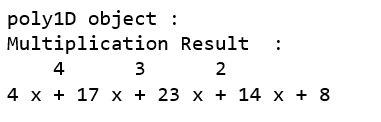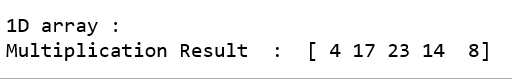# numpy.polymul() in Python

numpy.polymul(p1, p2) method evaluates the product of two polynomials and returns the polynomial resulting from the multiplication of two input polynomials ‘p1’ and ‘p2’.

Parameters :
p1 : [array_like or poly1D]Input polynomial 1.
p2 : [array_like or poly1D]Input polynomial 2.

Return: Polynomial resulting from multiplication of the inputs.

If either input is `poly1D `object, then the output is also a poly1D object otherwise, 1D array of polynomial coefficients in decreasing order of degree.

Code : Python code explaining polymul()

 `# Python code explaining  ` `# numpy.polymul() ` `   `  `# importing libraries ` `import` `numpy as np ` `import` `pandas as pd ` ` `  `# Constructing polynomial  ` `p1 ``=` `np.poly1d([``1``, ``2``])  ` `p2 ``=` `np.poly1d([``4``, ``9``, ``5``, ``4``])  ` `   `  `print` `(``"P1 : "``, p1)  ` `print` `(``"\n p2 : \n"``, p2)  ``mul ``=` `np.polymul(p2, p1) ` ` `  `print``(``"\n\npoly1D object : "``) ` `print``(``"Multiplication Result  : \n"``, mul) ``# Defining ndarray ` `x ``=` `np.array([``1``, ``2``]) ` `y ``=` `np.array([``4``, ``9``, ``5``, ``4``]) ` `mul ``=` `np.polymul(y, x) ` ` `  `print``(``"\n1D array : "``) ` `print``(``"Multiplication Result  : "``, mul) `My Personal Notes arrow_drop_upIf you like GeeksforGeeks and would like to contribute, you can also write an article using contribute.geeksforgeeks.org or mail your article to contribute@geeksforgeeks.org. See your article appearing on the GeeksforGeeks main page and help other Geeks.

Please Improve this article if you find anything incorrect by clicking on the "Improve Article" button below.

Article Tags :

Be the First to upvote.

Please write to us at contribute@geeksforgeeks.org to report any issue with the above content.